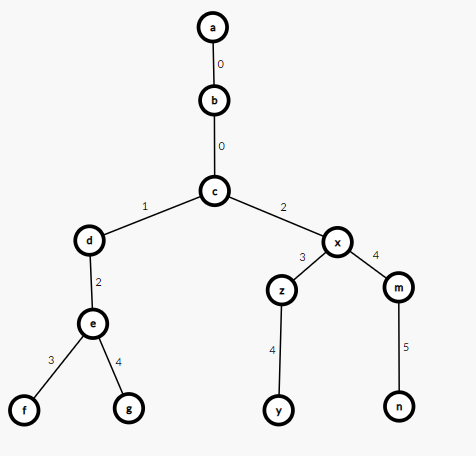### quanlt206's blog

By quanlt206, history, 14 months ago,## Problems

Give an array consists N integers and Q queries, with two types :

1. ? k -> print the kth element in this array.

2. ! k -> remove the kth element in this array.

a[i] <= 1e9 (1 <= i <= N) N, Q <= 2e5

This problem likes "List Removal" problems on Cses (here)

## Naive Approach

Using brute-force, query 1 will be processed in O(1), query 2 will be processed in O(n).

Code:

void Query_1(int k) {
cout<<a[k]<<" ";
}
void Query_2(int k) {
for (int i = k; i < n; i++) a[i] = a[i + 1];
n--;
}


## O(nlog(n)log(n)) / O(nlogn) using IT, BIT, ordered_set

Because using IT(Segment Tree) and BIT is the same, so now I'm talking about IT.

Firstly, we will have an array B, B[i] = 1 if ith element is not deleted yet, B[i] = 0 otherwise. We will build a Segment Tree whose each node has 1 value, it is the sum B[i] in [L, R].

Now, we can find the kth element in the array easily by binary search. Code:

int Find(int k) {
int l = 1, r = n, mid, res;
while (l <= r) {
mid = (l + r) >> 1;
if (GetSum(1, mid) >= k) {
res = mid;
r = mid - 1;
}
else l = mid + 1;
}
return res;
}


We can optimize the complexity from O(nlog(n)^2) to O(nlog(n)) using "Walk on segment tree" technique.

This problem can solve very easily by ordered_set with O(nlog(n)): https://www.geeksforgeeks.org/ordered-set-gnu-c-pbds/

## Using Trie, O(nlogn) complexity

This is for people who don't know Trie.

The main idea is inserting all index in array into Trie. Firstly, we will converse all index to string, and all string has the same length (we will insert "0" before some strings). Now the problem is find the Kth string (lexicographically) smallest in Trie.

We will build a Trie, each node like :

struct Node {
Node* child;
int cnt, val;
Node() {
for (int i = 0; i < 10; i++) child[i] = NULL;
cnt = val = 0;
}
};

• cnt : numbers of string pass this node
• val : if this Node is the end of a string (index), val = a[index].

Okay, now we will find the way to reach the Kth smallest string (lexicographically).

Consider we add some strings into Trie: "00123" "00124" "00234" "00245" And K = 4 => 00245

We will have Trie like :Assume we are in node a, there are 4 strings passed node b, we go to node b.

At node b, we see node c, it has 4 strings like b, we go to node c.

At node c, consider node d, it has 2 strings passed, so the Kth strings never passed this node, we decrease K by 2. Now, K = 2

At node c, consider node x, it has 2 strings passed, 2 >= K, so the Kth string passed this node, we go to node x.

At node x, consider node z, it has 1 string passed, but K = 2, so we decrease K by 1, K = 1.

At node x, consider node m, it has 1 string passed, we go to node m.

At node m, consider node n, it has 1 string passed, we go to node n.

Now, the path a -> b -> c -> x -> m -> n is the 4th smallest string, it is "00245".

Code:

string FindTheKthSmallestString(int k) {
Node* p = Trie_root;
string res = "";
while (true) {
bool kt = true;
for (int i = 0; i < 10; i++)
if (p->child[i] != NULL) {
if (k > p->child[i]->cnt) k-=p->child[i]->cnt; else {
res+=char(i + 48);
p = p->child[i];
kt = false;
break;
}
}
if (kt) break;
}
return res;
}



Now, we can use this technique to solve this problem.

This is my code for List Removals:

#include<bits/stdc++.h>
#define X first
#define Y second
#define all(x) begin(x), end(x)
#define FOR(i, a, b) for(int i = (a); i <= (b); i++)
#define FORD(i, b, a) for(int i = (b); i >= (a); i--)
#define REP(i, a, b) for (int i = (a); i < (b); i++)
#define mxx max_element
#define mnn min_element
#define SQR(x) (1LL * (x) * (x))

using namespace std;

typedef long long ll;
typedef double db;
typedef long double ld;
typedef pair<int, int> pii;
typedef pair<int, pii> piii;
typedef pair<ll, ll> pll;
typedef pair<ll, pll> plll;
typedef pair<ll, int> pli;

template<class A, class B>
void maximize(A& x, B y) {
if (x < y) x = y;
}
template<class A, class B>
void minimize(A& x, B y) {
if (x > y) x = y;
}
/* END OF TEMPLATE */

int n, x;

struct Node {
Node* child;
int cnt, val;
Node() {
REP(i, 0, 10) child[i] = NULL;
cnt = val = 0;
}
};
Node* root = new Node();

void add(string& s, int val) {
Node* p = root;
for (auto x : s) {
if (p->child[x - 48] == NULL) p->child[x-48] = new Node();
p = p->child[x - 48];
p->cnt++; // string passed
}
p->val = val;
}
// find and remove
int dfs(Node* p, int k) {
while (k > 0) {
bool kt = true;
REP(i, 0, 10)
if (p->child[i] != NULL) {
// if p->child[i]->cnt == 0, it were deleted.
if (p->child[i]->cnt !=0 && p->child[i]->cnt >= k) {
p->child[i]->cnt--;
p = p->child[i];
kt = false;
break;
}
else k-=p->child[i]->cnt;
}
if (kt) break;
}
return p->val;
}
int main() {
ios_base::sync_with_stdio(0);
cin.tie(0);
cin>>n;
FOR(i, 1, n) {
cin>>x;
string s = to_string(i);
while ((int)s.size() < 8) s = "0" + s; // make all string of index have the same length